ANTICIPATED SUSTAINED CONDITIONS CONSIDERING PIPE LIFT-OFF

# 1ANTICIPATED SUSTAINED CONDITIONS CONSIDERING PIPE LIFT-OFF

## 1.1 Problem definition

As same as in the first ASME-example (Chapter Fehler: Referenz nicht gefunden) a code complient analysis of a piping system is to be performed. Special consideration has to be given to the fact that one support lifts up during operation.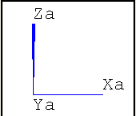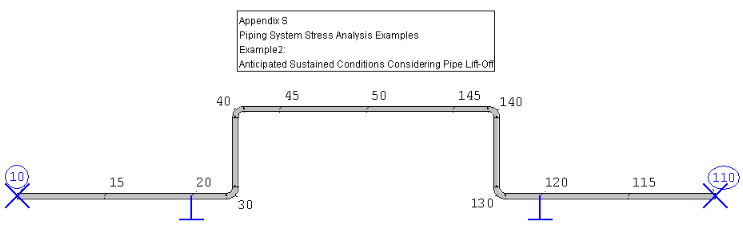Figure 2: Rohr2 Model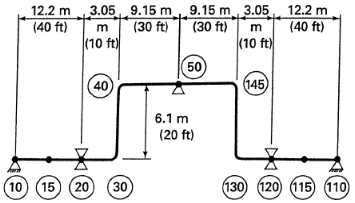Figure 1: Model by ASME

## 1.2 References (ASME B31.3)

ASME B31.3-2008 Process Piping (ASME Code for Pressure Piping, B31)

Revision of ASME B31.3-2006, The American Society of Mechanical Engineers, New York, NY, Appendix S, pg. 286-289

A beam system with design and operating conditions under the effect of gravity. The pipe components are defined by ASME. The following listed values are used: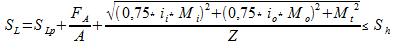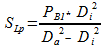Where:

 Variable Description Unit Used Value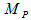Pipe material --- ASTM A 106 Grade B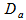Outside diameter (NPS) mm 406,4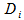Inside diameter mm 390.54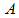Cross section mmÂ² 9933,15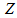Section Modulus mmÂ³ 970567,7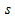Nominal wall thickness mm 9,53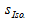Insulation thickness mm 127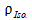Insulation density kg/mÂ³ 176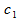Corrosion allowance mm 1,59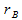Bend radius mm 609,5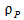Pipe density kg/mÂ³ 7833,4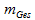Unit weight kg/m 248,3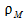Fluid specific gravity kg/mÂ³ 1000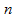Number of cycles --- < 7000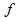Stress range factor --- 1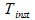Installation temperature Â°C 21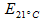Modulus of Elasticity N/mmÂ² 203010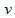Poisson's ratio --- 0,3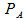Design pressure bar Ã¼ 39,68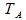Design temperature Â°C 302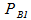Operating pressure1 bar Ã¼ 37,95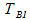Operating temperature1 Â°C 288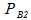Operating pressure2 bar Ã¼ 0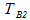Operating temperature2 Â°C -1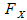Horizontal support loads N ---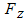Vertical support loads N ---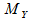Moments at supports Nm ---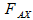Axial section force N ---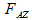Vertical section force N ---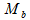Bending moment Nm ---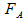Axial force N ---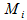Bending moment (In-Plane) Nm ---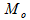Bending moment (Out-Plane) Nm ---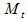Torsional moment Nm 0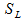Longitudinal stress N/mmÂ² ---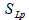Pressure induced stress N/mmÂ² 45,76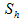Allowable stress at maximum metal temp. N/mmÂ² 130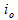stress intensification factor (Out-Plane) for branch --- 2,14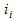stress intensification factor (In-Plane) for branch --- 2,57

Table 1: Overview of the used variables

## 1.3 Model description (ROHR2)

The known system geometry from the previous example is used. A few modifications must be taken into account. The this example consists of the same geometry as in the eleventh, but it is mirrored at the vertical axis. Hence of this, the calculated system is symmetric. The example has a total length of 61 meters. It consists of steel pipes (ASTM A 106 Grade B). The calculation model has four rigid anchors and two sliding supports. All supports have indefinite stiffness. The ASME example contaons a slide support at node 50 which lifts up. The ASME-Code regards several listed sustained load conditions, but it evaluates just one of them. In this case, the support at node 50 mustn't be attached. The following specific parameter are equal to the previous example:

• Characteristic material values

• Pipe density, Poisson's ratio, modulus of elasticity, mean coefficient of linear thermal expansion and basic allowable stresses

• Dimensions

• Corrosion allowances

• Insulation parameters

• Load cases and their properties

• Stress analysis conditions

A detailed list of the input parameters of the model is given in the table above.

## 1.4 Result comparisons

The following tables compare only two adequately points of each verification value. These results apply to the sustained load condition 3 (Appendix S, page 289) where the support status of node 50 had to be deactivated.All results are given in a global coordinate system. The whole comparison is shown in the document R2_stresses12.ods.

### 1.4.1 Results for operating case 1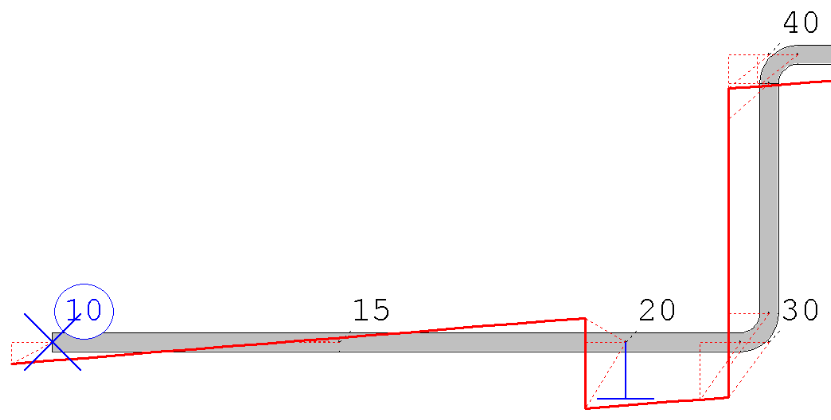Figure 4: ROHR2 model (detail) with force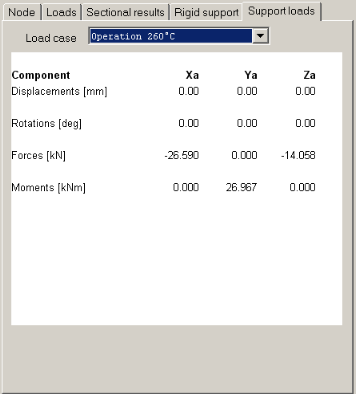Figure 3: Support loads, node 10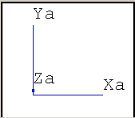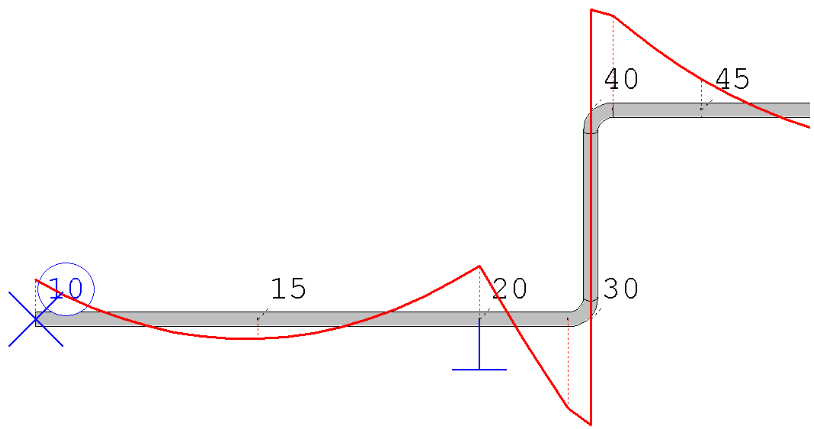Figure 6: ROHR2 model (detail) with force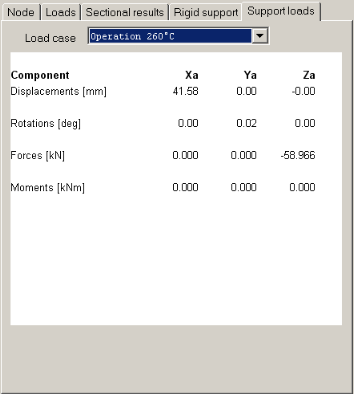Figure 5: Support loads, node 20

 Point Value Reference (ASME) [N] Rohr2 [N] Difference [%] 10-26600 -26590 <0,04

Table 2: Comparison of the horizontal support load for node 10

 Point Value Reference (ASME) [N] Rohr2 [N] Difference [%] 10-14050 -14058 <0,06 20 58900 -58966 <0,12

Table 3: Comparison of the vertical support load for node 10, 20

 Point Value Reference (ASME) [Nm] Rohr2 [Nm] Difference [%] 1027000 26967 <0,13

Table 12.4.1.3: Comparison of the moments at supports for node 10

### 1.4.2 Sustained load case results 1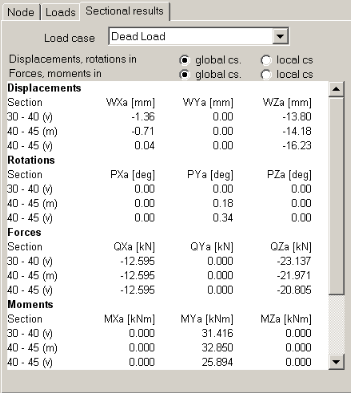Figure 7: sectional results, node 40m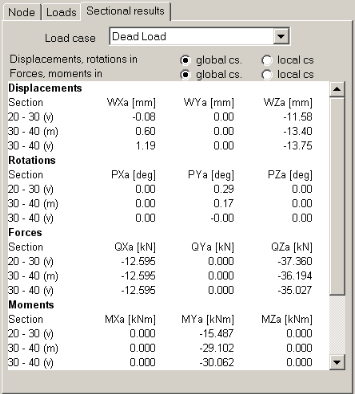Figure 8: sectional results of node 30n

 Point Value Reference (ASME) [N] Rohr2 [N] Difference [%] 30n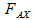12575 12595 <0,16 40m 12575 12595 <0,16

Table 4: Comparison of the axial section force for node 30n, 40m

 Point Value Reference (ASME) [N] Rohr2 [N] Difference [%] 30n34985 35027 <0,12 40m 21952 21971 <0.09

Table 5: Comparison of the vertical section force for node 30n, 40m

 Point Value Reference (ASME) [Nm] Rohr2 [Nm] Difference [%] 30n29985 30062 <0,26 40m 32770 32850 <0.25

Table 6: Comparison of the Bending Moment for node 30n, 40m

### 1.4.3 Sustained load case results 2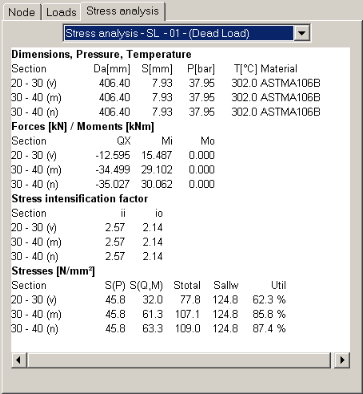Figure 9: SL results at node 30n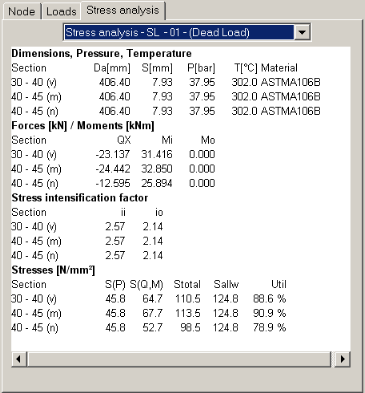Figure 10: SL results at node 40m

 Point Value Rohr2 [N] 30n-35027 40m 24442

Table 7: Converted axial force of node 30n, 40m

 Point Value Rohr2 [N] 30n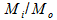30062 40m 32850

Table 8: Converted in or out-plane moments of node 30n, 40m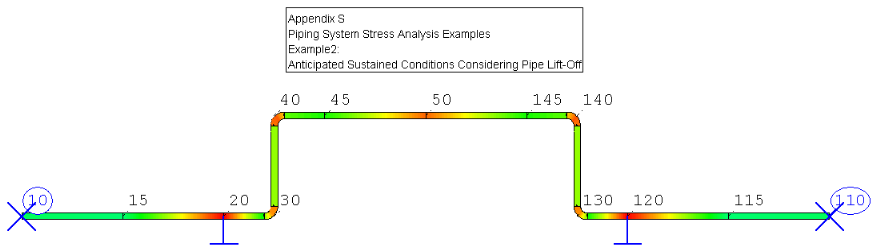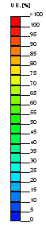Figure 11: ROHR2 model with SL-stresses

 Point Value Reference (ASME) [N/mmÂ²] Rohr2 [N/mmÂ²] Difference [%] 30n101,920 109,000 <6.5 40m 108,525 113,500 <4.4

Table 9: Comparison of the longitudinal stresses for node 30n, 40m

## 1.5 Conclusion

The results for all points are listed in the R2_stresses12.ods document. Only one half of the system was documented because of the pipe and load symmetry. The results are close to the reference. The reasons for the differences are the same as in the previous example.

## 1.6Files

R012_inch.r2w

R012_mm.r2w

R2_stresses12.ods

MATDAT.r2u

SL-Ber.Pkt.10.mcd

SL-Ber.Pkt.20.mcd

SL-Ber.Pkt.30n.mcd

SL-Ber.Pkt.40m.mcd

SL-Ber.Pkt.50.mcd

SIGMA Ingenieurgesellschaft mbH www.rohr2.com